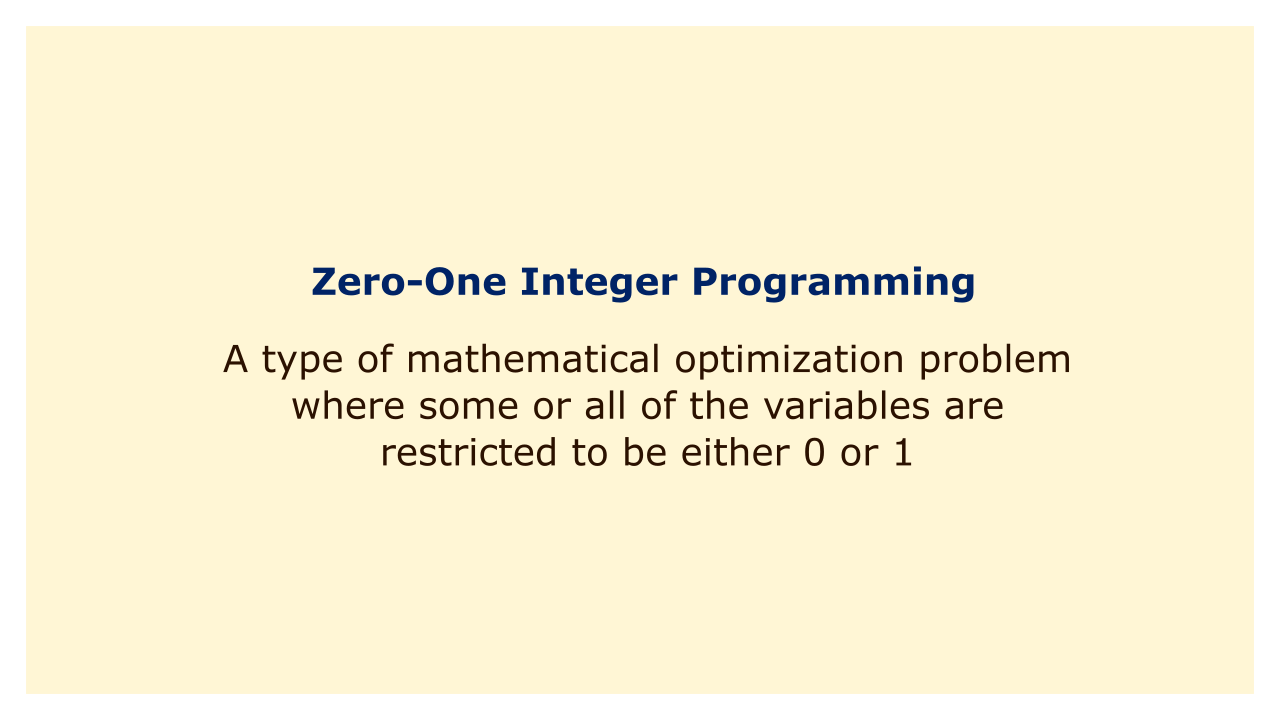# Zero-One Integer ProgrammingImage: Moneybestpal.com

### Zero-one integer programming is a type of mathematical optimization problem where some or all of the variables are restricted to be either 0 or 1.

Compared to normal integer programming problems, this indicates that the solution space is discrete and finite, making it simpler to solve. Nonetheless, zero-one integer programming issues can still be quite difficult, particularly when they contain a lot of variables and restrictions.

In combinatorial optimization, where the objective is to identify the optimum arrangement of a limited number of components that fulfills a set of requirements, zero-one integer programming is one of the techniques most frequently used. Let's take an example where you want to choose a subset of items from a larger collection while ensuring that their combined value is maximized and their combined weight does not go over a specific threshold. This is known as the knapsack problem, and it can be formulated as a zero-one integer programming problem as follows:

Let x_i be a binary variable that indicates whether item i is selected or not, where i = 1, 2, ..., n.

Let v_i be the value of item i, and w_i be the weight of item i.

Let W be the weight limit of the knapsack.

The objective function is to maximize the total value of the selected items:

maximize sum_{i=1}^n v_i x_i

The constraint is to ensure that the total weight of the selected items does not exceed the weight limit:

sum_{i=1}^n w_i x_i <= W

The binary restriction is to ensure that each item is either selected or not:

x_i in {0, 1} for all i = 1, 2, ..., n

Zero-one integer programming issues can be solved in a variety of ways, including branch and bound, cutting plane, branch and cut, etc. These techniques usually entail removing the binary restriction and solving a linear programming problem, followed by the application of certain rules to remove or refine the solution space until an optimal or nearly optimal solution is discovered.
Tags